D-error is a way of summarizing how good or bad a design is at extracting information from respondents in a choice experiment. A design with a low D-error is better than a design with a high D-error, provided that both designs are for the same experiment; comparing D-error between designs for different experiments is meaningless. Many other related measures exist that also serve this purpose, such as D-optimality.

This article gives an overview of D-error and demonstrates how to compute D-error by working through an example. Concepts in this article are covered in more (mathematical) detail here.

## Prior parameter assumptions

When computing D-error, a prior assumption about the respondent parameters needs to be made. D0-error assumes that all parameters are zero — i.e., respondents have no preference for any of the attribute levels. DP-error assumes that all respondent parameters are equal to a parameter vector. On the other hand, DB-error assumes that respondent parameters are distributed according to a probability distribution, which is often a multivariate normal distribution with a diagonal covariance matrix.

## DP-error example

A small choice experiment design is shown below:

1111121
1112212
1221122
1222211
1331221
1332112
2411222
2412111
2521222
2522111
2631121
2632212

The first step is to encode the design, with either dummy coding or effects coding. I use dummy coding in this example, and split the encoded design by its four questions: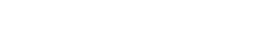For DP-error, I assume that the respondent parameters are given by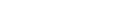. The next step is to compute the multinomial logit probabilities, using the formula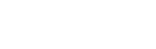where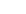refers to the probability of selecting alternative i out of J alternatives in question q. The probabilities are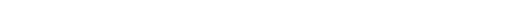These probabilities are then used to construct the Fisher information matrix, using the formula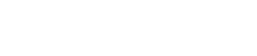Plugging the values for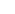and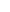into the formula, the information matrix isThe DP-error is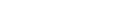, where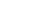is the number of parameters.

## D0-error example

Computing D0-error is just a special case of DP-error where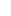is assumed to be a vector of zeros. In this case, the probabilities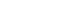(=1/2 in this example) and the information matrix isThe D0-error is.

## DB-error (Bayesian) Example

DB-error is defined as the integral of DP-error over an assumed prior distribution of the respondent parameters. One way to compute this numerically is known as Monte Carlo estimation. It involves calculating the average DP-error of many sets of parameters randomly drawn from the prior distribution. To illustrate this, assume that the parameter distribution is multivariate normal with meanand a covariance matrix that is diagonal with standard deviations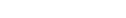. I draw 1000 samples from this distribution, as partially shown in the table below:

DrawParameter 1Parameter 2Parameter 3Dp-error
10.25-0.730.671.45
21.14-0.670.671.90
30.69-0.501.231.92
...............
10001.15-1.670.572.82

DB-error is estimated as the mean DP-error, which is 1.90 in this example. Another more computationally efficient but complicated way of computing the integral using quadrature exists1 but is beyond the scope of this article.

Read more handy How To guides, or check out the rest of our blog!